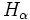# Difference between revisions of "Ascendant subgroup"

This article defines a subgroup property: a property that can be evaluated to true/false given a group and a subgroup thereof, invariant under subgroup equivalence. View a complete list of subgroup properties[SHOW MORE]
If the ambient group is a finite group, this property is equivalent to the property: subnormal subgroup
View other properties finitarily equivalent to subnormal subgroup | View other variations of subnormal subgroup |
This is a variation of subnormality|Find other variations of subnormality |

## Definition

A subgroup$H$ of a group$G$ is termed ascendant if we have subgroups$H_\alpha$ of$G$ for every ordinal$\alpha$ such that:

•$H_0 = H$
•$H_\alpha \ \underline{\triangleleft} \ H_{\alpha + 1}$ (viz$H_\alpha$ is a normal subgroup of$H_{\alpha + 1}$) for every ordinal$\alpha$.
• If$\alpha$ is a limit ordinal, then$H_\alpha = \bigcup_{\gamma < \alpha} H_\gamma$, i.e., it is the union of all preceding subgroups. Note that the union of any ascending chain of subgroups is indeed a sugbroup (in fact, more generally, directed union of subgroups is subgroup). We can also define$H_{\alpha}$as$\langle H_\gamma \rangle_{\gamma < \alpha}$, i.e., it is the join of all preceding subgroups.

and such that there is some ordinal$\beta$ such that$H_\beta = G$.

### In terms of the ascendant closure operator

The subgroup property of being an ascendant subgroup is obtained by applying the ascendant closure operator to the subgroup property of being normal.

## Relation with other properties

### Stronger properties

Property Meaning Proof of implication Proof of strictness (reverse implication failure) Intermediate notions
normal subgroup we can get a series that reaches the group in one step.
subnormal subgroup we can get a series that reaches the group in finitely many steps. (obvious) ascendant not implies subnormal |FULL LIST, MORE INFO
hypernormalized subgroup we can use the series where the subgroup for each successor ordinal is the normalizer in the whole group of the subgroup for the ordinal. (obvious) |FULL LIST, MORE INFO

### Weaker properties

Property Meaning Proof of implication Proof of strictness (reverse implication failure) Intermediate notions
serial subgroup

## Metaproperties

Metaproperty name Satisfied? Proof Statement with symbols
transitive subgroup property Yes ascendance is transitive If$H \le K \le G$ are groups such that$H$ is an ascendant subgroup of$K$ and$K$ is an ascendant subgroup of$G$, then$H$ is an ascendant subgroup of$G$.
trim subgroup property Yes Every group is ascendant in itself, and the trivial subgroup is ascendant in any group.
intermediate subgroup condition Yes ascendance satisfies intermediate subgroup condition If$H \le K \le G$ are groups such that$H$ is an ascendant subgroup of$G$, then$H$ is an ascendant subgroup of$K$.
strongly intersection-closed subgroup property Yes ascendance is strongly intersection-closed If$H_i, i \in I$, are all ascendant subgroups of a group$G$, so is$\bigcap_{i \in I} H_i$.
transfer condition Yes ascendance satisfies transfer condition If$H,K \le G$ are subgroups and$H$ is ascendant in$G$, then$H \cap K$ is ascendant in$K$.
image condition Yes ascendance satisfies image condition Suppose$H$ is an ascendant subgroup of a group$G$, and$\varphi:G \to K$ is a surjective homomorphism of groups. Then,$\varphi(H)$ is an ascendant subgroup of$K$.